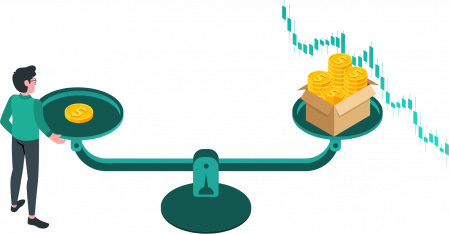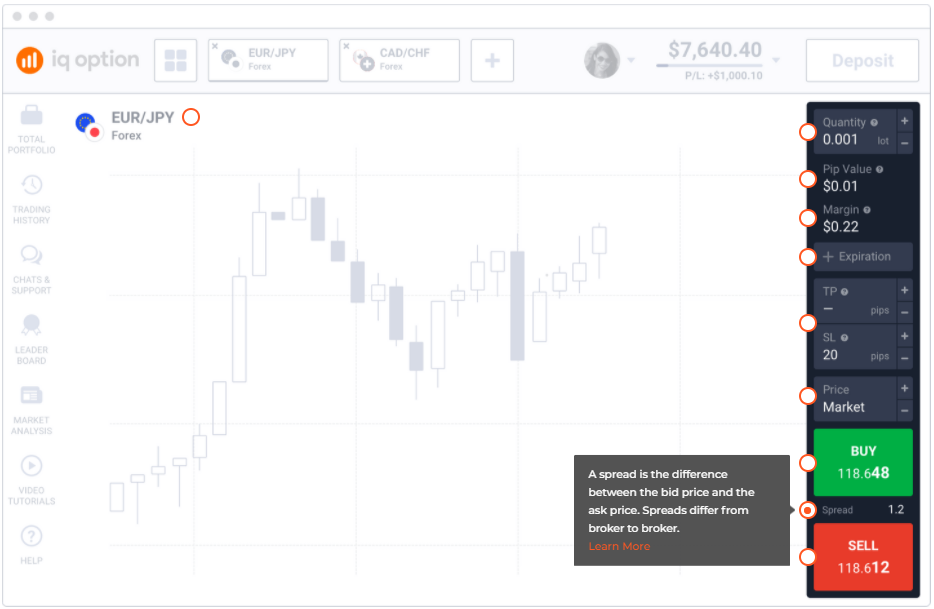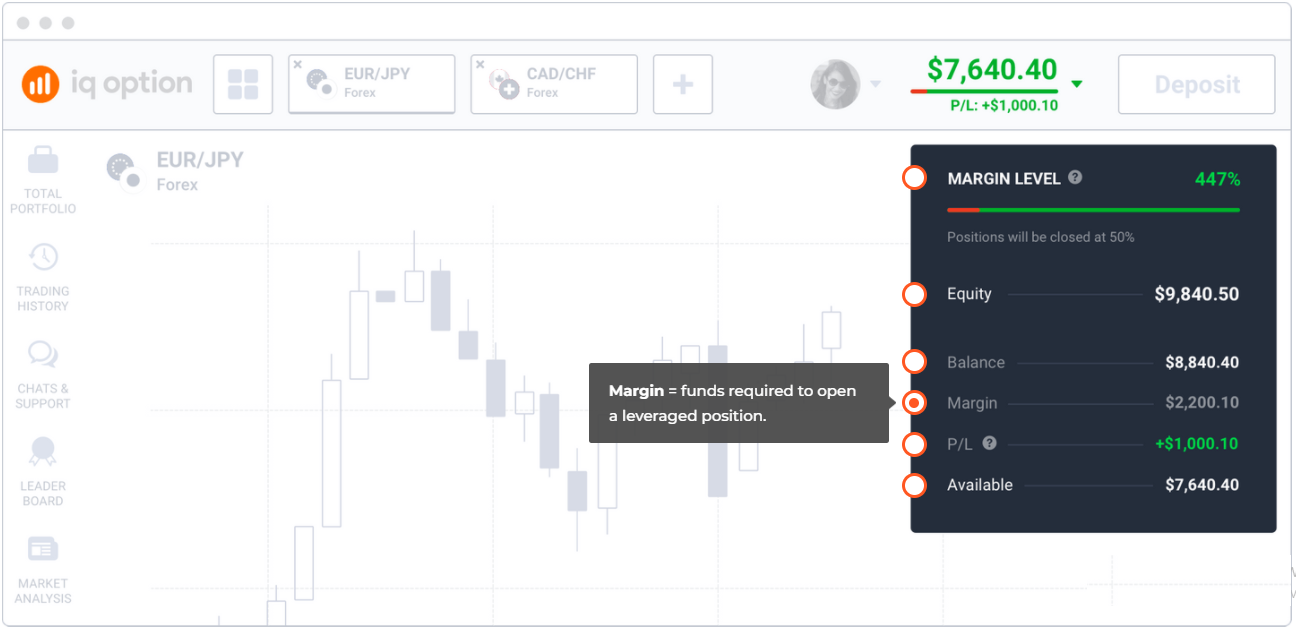# The Basics Trading in IQ Option: Spreads, Swaps, Margin, Leverage, ConversionsA spread is the difference between the bid price and the ask price. Spreads differ from broker to broker.
To calculate the cost of a spread on the IQ Option platform, use the following formula:

Cost of a spread = Lot size × Contract size × Spread

Example
Spread: 1.13462 – 1.13455 = 0.00007
Contract size: 100.000 units of the base currency (=200,000 EUR)
EUR/USD cost of spread = (1.13462 – 1.13455) × 2 × 100.000 = 14 USD## Swaps

A swap is an interest charge that a trader has to pay to a broker for holding positions overnight.

Swaps arise from the difference in interest rates of currencies plus the broker’s administrative fee. In forex trading, you borrow one currency in order to buy another. A swap depends on whether you buy a currency with a higher or lower interest rate compared to that of the currency you borrow. Swaps can be positive and negative.

If you buy a currency with a higher interest rate than that of the borrowed one, you will receive a positive swap. Let’s look at the following example.

Example
The American interest rate is 1.75%.
Australia’s interest rate is 0.75%.
If you open a long position on the USD/AUD pair, a swap of 0.75% will be credited to your account, as the currency you buy (USD) has a higher interest rate than the currency you borrow (AUD).
If you open a short position on the same currency pair, a swap of 1.25% will be debited from your account, because the currency you borrow (USD) has a higher interest rate than the currency you buy (AUD).

## Margin

Margin is the amount of a trader’s funds required to open a leveraged position. Margin allows you to trade with leverage, which is essentially using borrowed funds from a broker in order to increase the size of your trades.
To calculate a margin on the IQ Option platform, use the following formula:

Margin = Lot size × Contract size / Leverage

Example
You intend to buy 0.001 lots (1,000 units) of the EUR/USD currency pair with a 1:500 leverage. The margin required to open this trading position is 0.2 EUR. Check out the detailed calculations below:

Currency pair: EUR/USD
Lot size: 0.001 lot
Leverage: 1:500
Contract size: 100,000 units of the base currency
Margin = 0.001 × 100,000 / 500 = 0.2 EUR
Please note that conversion may apply if your account currency differs from the base currency.

## Leverage

Leverage allows you to trade positions larger than the amount of capital you possess. Leverage maximizes payouts, but it also maximizes losses.

Example
Let’s assume you have deposited \$1,000 into your account and are using a 1:500 leverage. In this case, your buying power will increase by 500 times, to \$500,000, which means you can place a trade with a value of \$500,000.
Please note that leverage varies for different assets.

## Conversions

Currency conversion rates may apply in some cases. This occurs due to the fact that each parameter of a trade is denominated either in the base currency or quote currency. A contract size and margin are denominated in the base currency, while payout is always calculated in the quote currency. Hence currency conversion rates may apply for calculating margin and payouts. If your account currency differs from the quote currency, conversions will apply. Let’s look at the following examples to understand when currency conversion may be required.

Example 1: Base currency = account currency

Let’s assume that your account currency is USD and you are trading the USD/JPY currency pair. Conversion will not apply when calculating the margin, as the base currency (USD) is the same as the account currency (USD). Conversion will apply when calculating the payout: first, it will be calculated in JPY, the quote currency, and then converted into USD, the account currency.

Example 2: Quote currency = account currency

Let’s assume that your account currency is USD and you are trading the EUR/USD currency pair. Conversion will apply when calculating the margin, as the base currency (EUR) differs from the account currency (USD). Conversion won’t apply when calculating payouts, because the quote currency (USD) is the same as the account currency (USD).

Example 3: No matches

Let’s assume that your account currency is GBP and you are trading the AUD/CHF currency pair. Conversion will apply when calculating the margin, because the account currency (GBP) differs from the base currency (AUD). Conversion will also apply when calculating payouts: first, it will be calculated in CHF, the quote currency, and then converted into GBP, the account currency.

## Margin level

Margin level helps you monitor your account health: it shows whether everything is going well or not and suggests when you should close positions that are not profitable.
To calculate your margin level, use the following formula:

Margin level = Equity / Margin × 100%

Everything is indicated in the account currency:## Margin call and Stop out

### Margin call

When a trader’s margin level falls below 100%, the broker initiates a procedure known as a margin call. In the event of a margin call, the trader is required to either deposit more money into his/her account or close losing positions. If the margin level falls below 50%, losing positions will be forcibly closed by the company.

### Maintenance margin

Maintenance margin is the minimum amount of capital a trader must have in his or her account in order to keep a leveraged position open.

### Stop out

A stop out is an event that occurs when a trader’s equity is not sufficient to maintain open positions, hence they get forcibly closed by the broker.
Thank you for rating.